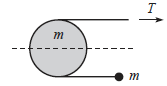# Rotational MechanicsA solid cylinder of mass $m$ and radius $r$ lies flat on friction less horizontal table, with a mass less string running halfway around it, as shown in figure. An object also of mass $m$ is attached to one end of the string, and you pull on the other end with a force $T$. The circumference of the cylinder is sufficiently rough so that the string does not slip with respect to it. What is the acceleration of the mass m attached to the end of the string?

Note: Give your answer with magnitude and direction. Choose right side to be positive and left side to be negative.

×